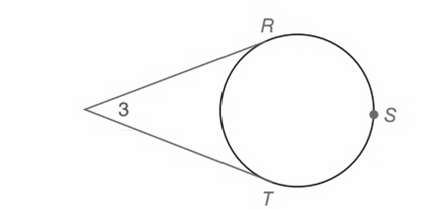Chapter 6.CT, Problem 7CTElementary Geometry For College St...

7th Edition
Alexander + 2 others
ISBN: 9781337614085

Solutions

Chapter
SectionElementary Geometry For College St...

7th Edition
Alexander + 2 others
ISBN: 9781337614085
Textbook Problem

Given the tangents with m ∠ 3 = 46 ° , find:a) m R S T ⌢ b) m R T ⌢To determine

(a)

To find:

mRST.

Explanation

Theorem used:

The measure of an angle formed when two secants intersect at a point outside the circle is one-half the difference of the measure of the two intercepts arcs.

Given:

m3=46°.

Calculation:

Consider the figure,

From the theorem we know that,

m3=mRSTmRT2(1).

Let us consider, mRST=x°.

And mRT=360°x°

Substitute for mRST and mRT in (1) we have,

To determine

(b)

To find:

mRT.

Still sussing out bartleby?

Check out a sample textbook solution.

See a sample solution

The Solution to Your Study Problems

Bartleby provides explanations to thousands of textbook problems written by our experts, many with advanced degrees!

Get Started

A sample with a mean of M = 8 has X = 56. How many scores are in the sample?

Essentials of Statistics for The Behavioral Sciences (MindTap Course List)

There exists a function f such that f(x) 0, f(x) 0, and f(x) 0 for all x.

Single Variable Calculus: Early Transcendentals, Volume I

Finding Derivatives In Exercises 51-62, find f(x). f(x)=x(x2+1)

Calculus: An Applied Approach (MindTap Course List)

Evaluate the integral. 36. 1+sinx1+cosxdx

Single Variable Calculus: Early Transcendentals

For 0

Study Guide for Stewart's Multivariable Calculus, 8th

True or False: If f′(c) = 0 and f″(c) < 0, then c is a local minimum.

Study Guide for Stewart's Single Variable Calculus: Early Transcendentals, 8th